# Thermodynamic integration

Thermodynamic integration is used to calculate the difference in the Helmholtz energy function,$A$, between two states. The path must be continuous and reversible, i.e., the system must evolve through a succession of equilibrium states (Ref. 1 Eq. 3.5)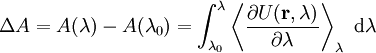$\Delta A = A(\lambda) - A(\lambda_0) = \int_{\lambda_0}^{\lambda} \left\langle \frac{\partial U(\mathbf{r},\lambda)}{\partial \lambda} \right\rangle_{\lambda} ~\mathrm{d}\lambda$

## Isothermal integration

At constant temperature (Ref. 2 Eq. 5):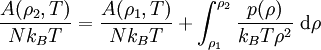$\frac{A(\rho_2,T)}{Nk_BT} = \frac{A(\rho_1,T)}{Nk_BT} + \int_{\rho_1}^{\rho_2} \frac{p(\rho)}{k_B T \rho^2} ~\mathrm{d}\rho$

## Isobaric integration

At constant pressure (Ref. 2 Eq. 6):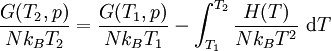$\frac{G(T_2,p)}{Nk_BT_2} = \frac{G(T_1,p)}{Nk_BT_1} - \int_{T_1}^{T_2} \frac{H(T)}{Nk_BT^2} ~\mathrm{d}T$

where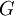$G$ is the Gibbs energy function and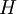$H$ is the enthalpy.

## Isochoric integration

At constant volume (Ref. 2 Eq. 7):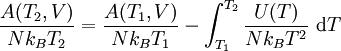$\frac{A(T_2,V)}{Nk_BT_2} = \frac{A(T_1,V)}{Nk_BT_1} - \int_{T_1}^{T_2} \frac{U(T)}{Nk_BT^2} ~\mathrm{d}T$

where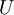$U$ is the internal energy.